# Recent Activity

## Sub-atomic product of funcoids is a categorical product ★★

Author(s):

Conjecture   In the category of continuous funcoids (defined similarly to the category of topological spaces) the following is a direct categorical product:
\item Product morphism is defined similarly to the category of topological spaces. \item Product object is the sub-atomic product. \item Projections are sub-atomic projections.

See details, exact definitions, and attempted proofs here.

Keywords:

## Bounding the on-line choice number in terms of the choice number ★★

Author(s): Zhu

Question   Are there graphs for whichis arbitrarily large?

## Are almost all graphs determined by their spectrum? ★★★

Author(s):

Problem   Are almost all graphs uniquely determined by the spectrum of their adjacency matrix?

Keywords: cospectral; graph invariant; spectrum

## Signing a graph to have small magnitude eigenvalues ★★

Author(s): Bilu; Linial

Conjecture   Ifis the adjacency matrix of a-regular graph, then there is a symmetric signing of(i.e. replace some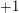entries by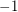) so that the resulting matrix has all eigenvalues of magnitude at most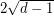.

Keywords: eigenvalue; expander; Ramanujan graph; signed graph; signing

## The Bollobás-Eldridge-Catlin Conjecture on graph packing ★★★

Author(s):

Conjecture  (BEC-conjecture)   Ifandare-vertex graphs and, thenandpack.

Keywords: graph packing

## Decomposing k-arc-strong tournament into k spanning strong digraphs ★★

Author(s): Bang-Jensen; Yeo

Conjecture   Every k-arc-strong tournament decomposes into k spanning strong digraphs.

Keywords:

## PTAS for feedback arc set in tournaments ★★

Author(s): Ailon; Alon

Question   Is there a polynomial time approximation scheme for the feedback arc set problem for the class of tournaments?

Keywords: feedback arc set; PTAS; tournament

## Partitionning a tournament into k-strongly connected subtournaments. ★★

Author(s): Thomassen

Problem   Letbe positve integer Does there exists an integer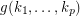such that every-strong tournamentadmits a partition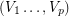of its vertex set such that the subtournament induced byis a non-trivial-strong for all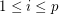.

Keywords:

## Weighted colouring of hexagonal graphs. ★★

Author(s): McDiarmid; Reed

Conjecture   There is an absolute constantsuch that for every hexagonal graphand vertex weighting,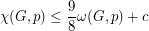Keywords:

## Colouring the square of a planar graph ★★

Author(s): Wegner

Conjecture   Letbe a planar graph of maximum degree. The chromatic number of its square is
\item at most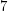if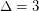, \item at mostif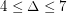, \item at most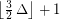if.

Keywords:

## List chromatic number and maximum degree of bipartite graphs ★★

Author(s): Alon

Conjecture   There is a constantsuch that the list chromatic number of any bipartite graphof maximum degreeis at most.

Keywords:

## Hamilton decomposition of prisms over 3-connected cubic planar graphs ★★

Author(s): Alspach; Rosenfeld

Conjecture   Every prism over a-connected cubic planar graph can be decomposed into two Hamilton cycles.

Keywords:

## Turán's problem for hypergraphs ★★

Author(s): Turan

Conjecture   Every simple-uniform hypergraph on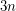vertices which contains no complete-uniform hypergraph on four vertices has at mosthyperedges.
Conjecture   Every simple-uniform hypergraph on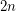vertices which contains no complete-uniform hypergraph on five vertices has at most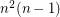hyperedges.

Keywords:

## 4-connected graphs are not uniquely hamiltonian ★★

Author(s): Fleischner

Conjecture   Every-connected graph with a Hamilton cycle has a second Hamilton cycle.

Keywords:

## Every prism over a 3-connected planar graph is hamiltonian. ★★

Author(s): Kaiser; Král; Rosenfeld; Ryjácek; Voss

Conjecture   Ifis a-connected planar graph, thenhas a Hamilton cycle.

Keywords:

## Hoàng-Reed Conjecture ★★★

Author(s): Hoang; Reed

Conjecture   Every digraph in which each vertex has outdegree at leastcontainsdirected cycles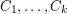such that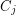meetsin at most one vertex,.

Keywords:

## Edge-disjoint Hamilton cycles in highly strongly connected tournaments. ★★

Author(s): Thomassen

Conjecture   For every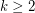, there is an integerso that every strongly-connected tournament hasedge-disjoint Hamilton cycles.

Keywords:

## Hamilton cycle in small d-diregular graphs ★★

Author(s): Jackson

An directed graph is-diregular if every vertex has indegree and outdegree at least.

Conjecture   For, every-diregular oriented graph on at mostvertices has a Hamilton cycle.

Keywords:

## Switching reconstruction of digraphs ★★

Author(s): Bondy; Mercier

Question   Are there any switching-nonreconstructible digraphs on twelve or more vertices?

Keywords:

## Switching reconstruction conjecture ★★

Author(s): Stanley

Conjecture   Every simple graph on five or more vertices is switching-reconstructible.

Keywords: reconstruction